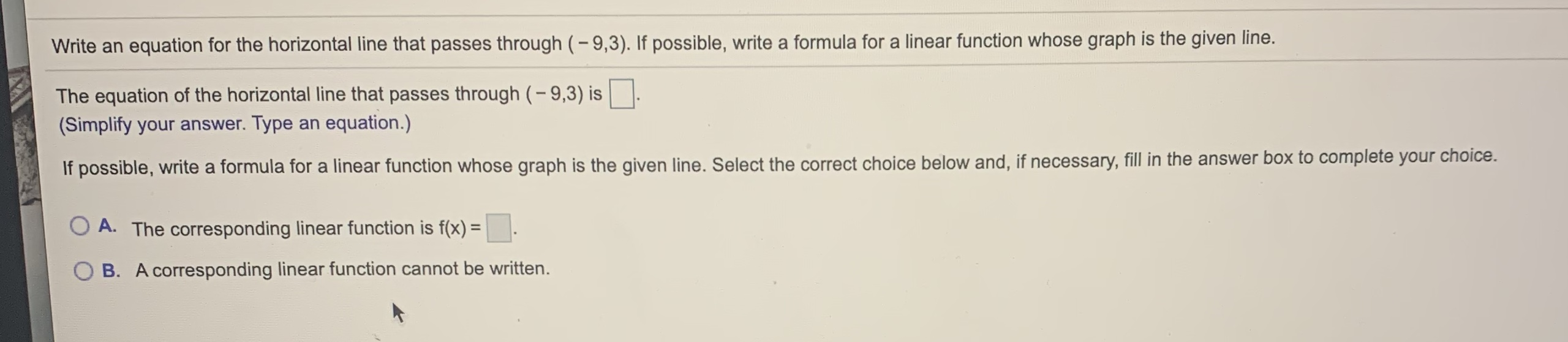# Write an equation for the horizontal line that passes through (-9,3). If possible, write a formula for a linear function whose graph is the given line. The equation of the horizontal line that passes through (- 9,3) is (Simplify your answer. Type an equation.) If possible, write a formula for a linear function whose graph is the given line. Select the correct choice below and, if necessary, fill in the answer box to complete your choice. A. The corresponding linear function is f(x) = B. A corresponding linear function cannot be written.

Questionhelp_outlineImage TranscriptioncloseWrite an equation for the horizontal line that passes through (-9,3). If possible, write a formula for a linear function whose graph is the given line. The equation of the horizontal line that passes through (- 9,3) is (Simplify your answer. Type an equation.) If possible, write a formula for a linear function whose graph is the given line. Select the correct choice below and, if necessary, fill in the answer box to complete your choice. A. The corresponding linear function is f(x) = B. A corresponding linear function cannot be written. fullscreen

### Want to see this answer and more?

Experts are waiting 24/7 to provide step-by-step solutions in as fast as 30 minutes!*

*Response times may vary by subject and question complexity. Median response time is 34 minutes for paid subscribers and may be longer for promotional offers.
Tagged in
Math
Algebra

### Equations and In-equations